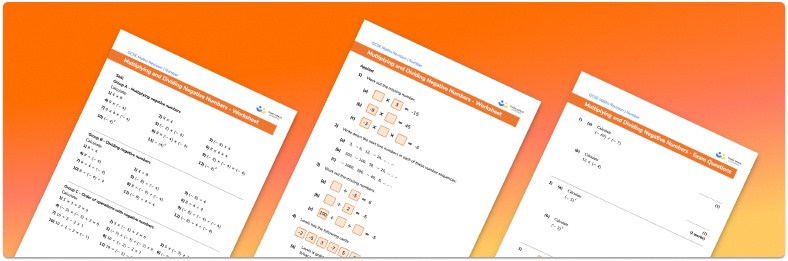# Multiplying Negative Numbers Worksheet• Section 1 of the multiplying negative numbers worksheet contains 20+ skills-based multiplying negative numbers questions, in 3 groups to support differentiation
• Section 2 contains 4 applied multiplying negative numbers questions with a mix of worded problems and deeper problem solving questions
• Section 3 contains 4 foundation and higher level GCSE exam style multiplying negative numbers questions
• Answers and a mark scheme for all multiplying negative numbers questions are provided
• Questions follow variation theory with plenty of opportunities for students to work independently at their own level
• All questions created by fully qualified expert secondary maths teachers
• Suitable for GCSE maths revision for AQA, OCR and Edexcel exam boards

• This field is for validation purposes and should be left unchanged.

You can unsubscribe at any time (each email we send will contain an easy way to unsubscribe). To find out more about how we use your data, see our privacy policy.

### Multiplying negative numbers a glance

Numbers greater than zero are called positive numbers and numbers less than zero are called negative numbers. Negative numbers are represented below 0 on a number line and are denoted by a negative sign in front of the number.

Positive and negative numbers can be represented on a number line and we can perform addition, subtraction, multiplication and division calculations with them. When multiplying and dividing negative numbers, there are specific rules that tell us whether the answer will be positive or negative. A negative number multiplied by a negative number gives a positive answer and a negative number multiplied by a positive number gives a negative answer. The same rules apply for division. To multiply or divide negative integers we work as if we are multiplying or dividing integers that are positive and then use the rules to decide whether the final answer should be positive or negative.

When calculating with negative numbers, we must always apply BIDMAS to determine the correct order of operations.

Looking forward, students can then progress to additional number worksheets, for example aor aFor more teaching and learning support on Number our GCSE maths lessons provide step by step support for all GCSE maths concepts.

## Do you have KS4 students who need more focused attention to succeed at GCSE?There will be students in your class who require individual attention to help them succeed in their maths GCSEs. In a class of 30, it’s not always easy to provide.

Help your students feel confident with exam-style questions and the strategies they’ll need to answer them correctly with our dedicated GCSE maths revision programme.

Lessons are selected to provide support where each student needs it most, and specially-trained GCSE maths tutors adapt the pitch and pace of each lesson. This ensures a personalised revision programme that raises grades and boosts confidence.

Find out more